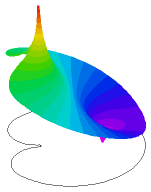﻿ Sample Problems > Applications > Electricity > space_charge

# space_charge

Navigation:  Sample Problems > Applications > Electricity >

# space_charge{  SPACE_CHARGE.PDE

This problem describes the electric field in an insulated cardioid-like

chamber due to an electrode at the tip of the cardioid and a localized

space charge near the center of the body.

Adaptive grid refinement detects the space charge and refines

the computation mesh to resolve its shape.

}

title "Electrostatic Potential with probe and space charge"

 select errlim = 1e-4 definitions  bigr = 1  smallr = 0.4  x0 = sqrt(bigr^2/2)  y0 = x0  r = sqrt(x^2+y^2) { define the electrode center }  xc = sqrt((bigr-smallr)^2/2)  yc = xc { A space charge source at -xc }  source = x/((x+xc)^2 + y^2 + 0.001)  k=0.1 variables  V equations  V : div(k*grad(V)) + source = 0 boundaries region 1   start(xc,yc-smallr)natural(V) = 0     { -- insulated outer boundary }

arc(center=xc,yc) to (x0,y0)

arc(center=0,0) angle 270

arc(center=xc,-yc) to (xc,smallr-yc)

value(V)=1         { -- applied voltage = 1 on tip }

arc(center=xc,0) angle -180 to close

plots

grid(x,y)

contour(V) as "Potential"

contour(V) zoom(0.2,-0.2,0.4,0.4)

surface(V) viewpoint (0,10,30)

surface(V) zoom(-0.6,-0.2,0.4,0.4)

surface(source) zoom(-0.6,-0.2,0.4,0.4)

end